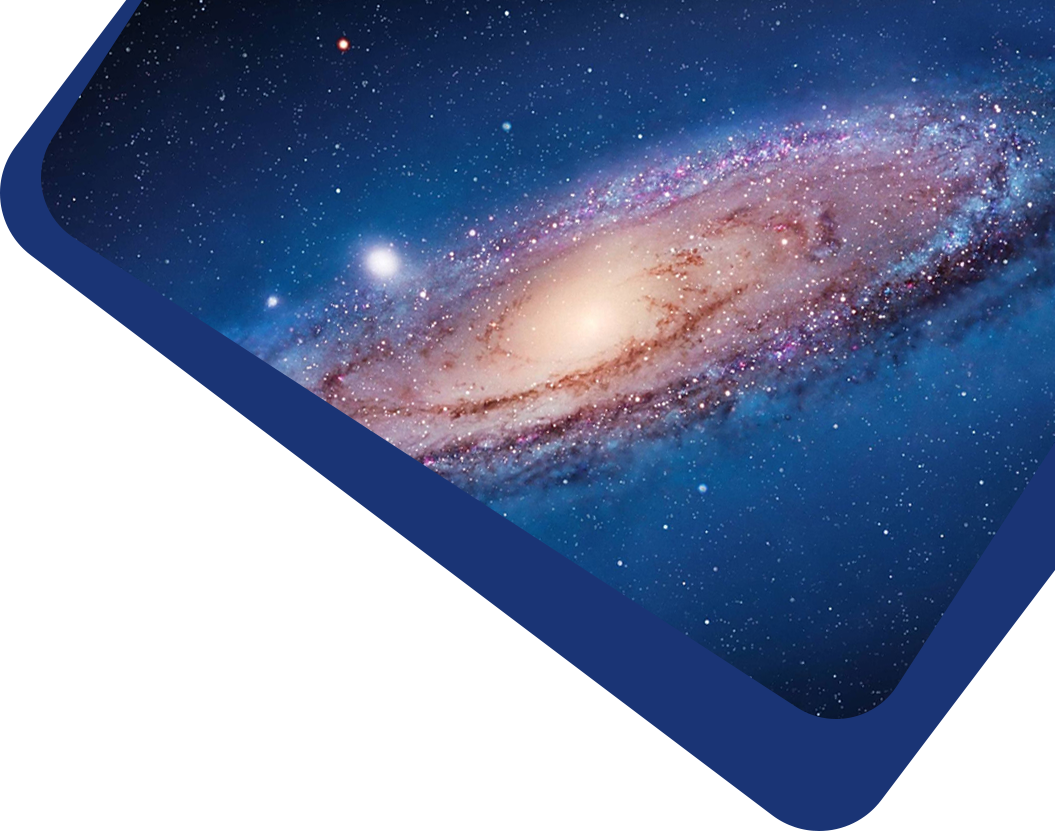Vol 19, No 4

## Stability of LRS Bianchi type-I cosmological models in f (R, T )-gravity

#### Umesh Sharma, Rashid Zia, Anirudh Pradhan, Aroon Beesham

Abstract

Abstract This paper examines the stability of the transition from the early decelerating stage of the Universe to the recent accelerating stage for the perfect fluid cosmological locally rotationally symmetric (LRS) Bianchi-I model in f(R,T) theory. To determine the solution of field equations, the idea of a time-varying deceleration parameter (DP) which yields a scale factor, for which the Universe attains a phase transition scenario and is consistent with recent cosmological observations, is used. The time-dependent DP yields a scale factor $$a = \exp [\frac{1}{β}\sqrt{2βt + k}]$$, where β and k are respectively arbitrary and integration constants. By using the recent constraints (H0 = 73.8, and q0 = −0.54) from Type Ia Supernova (SN Ia) data in combination with Baryonic Acoustic Oscillations (BAO) and Cosmic Microwave Background (CMB) observations (Giostri et al.), we obtain the values of β = 0.0062 and k = 0.000016 for which we have derived a cosmological model from the early decelerated phase to the present accelerating phase. By applying other recent constraints (H0 = 73.8, q0 = −0.73) from SNe Ia Union data (Cunha), we obtain the values of β = 0.0036 and k = 0.000084 for which we have derived a cosmological model in the accelerating phase only. We have compared both models with experimental data. The stability of the background solution has been examined also for the metric perturbations alongside the properties of future singularities in a Universe ruled by dark energy with phantom type fluid. We demonstrate the presence of a stable fixed point with a condition of state ω < −1 and numerically affirm this is really a late-time attractor in the ghost overwhelmed Universe. Some physical and geometric properties of the model are found and examined.

Keywords

Keywords LRS Bianchi-I Universe — Time-dependent deceleration parameter — f (R, T ) gravity theory — Transit universe

Full Text
Refbacks

• There are currently no refbacks.